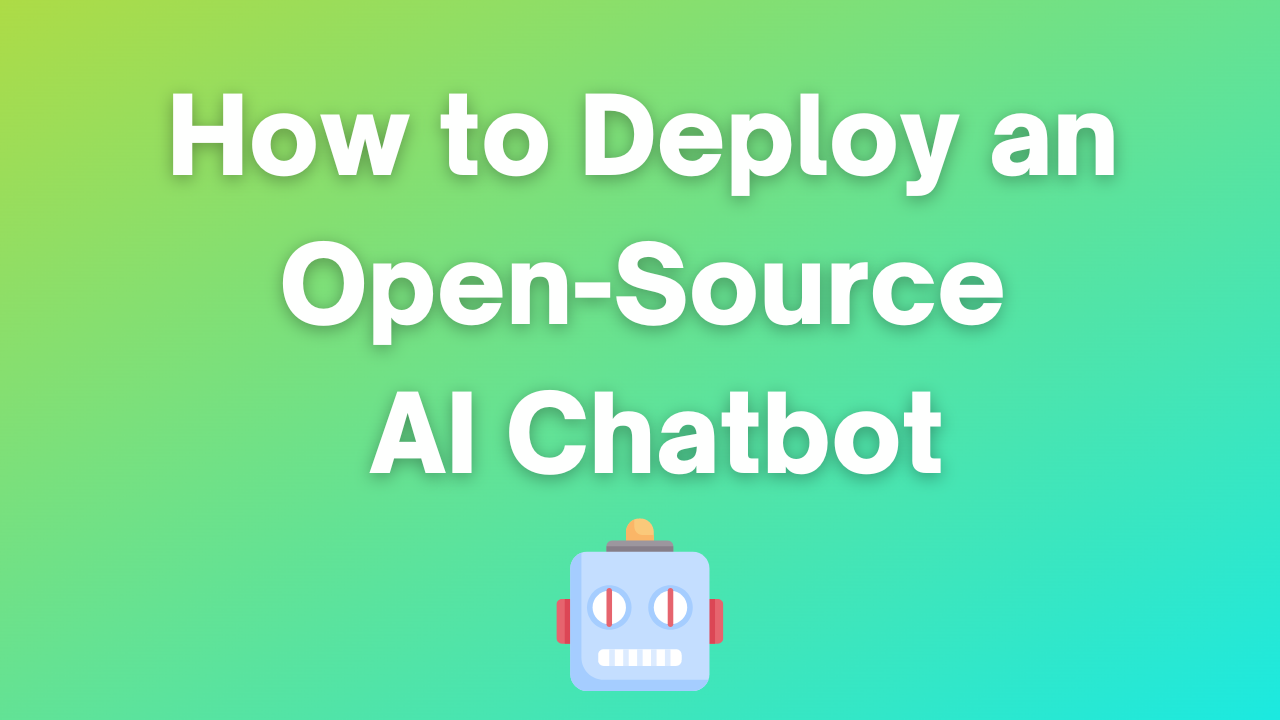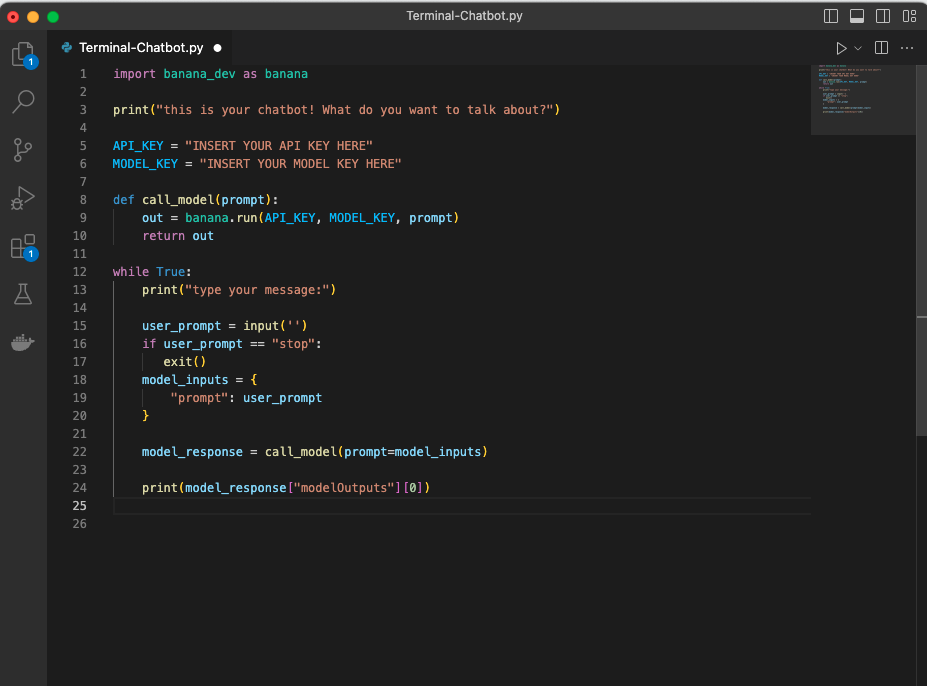How to Run an Open-Source AI Chatbot (not GPT-4 or ChatGPT!)

# How to Run an Open-Source AI Chatbot (not GPT-4 or ChatGPT!)## AI Chatbot Code Sample

Copy and paste the code below. Make sure your formatting matches the screenshot shown here:```import banana_dev as banana print("this is your chatbot! What do you want to talk about?") API_KEY = "INSERT YOUR API KEY HERE"```

`MODEL_KEY = "INSERT YOUR MODEL KEY HERE"`

``` def call_model(prompt): ```

`out = banana.run(API_KEY, MODEL_KEY, prompt) `

`return out`

``` while True: ```

```print("type your message:") user_prompt = input('') ```

` `

`if user_prompt == "stop": `

`exit() `

`model_inputs = { `

`"prompt": user_prompt `

`} `

```model_response = call_model(prompt=model_inputs) ```

`print(model_response["modelOutputs"])`